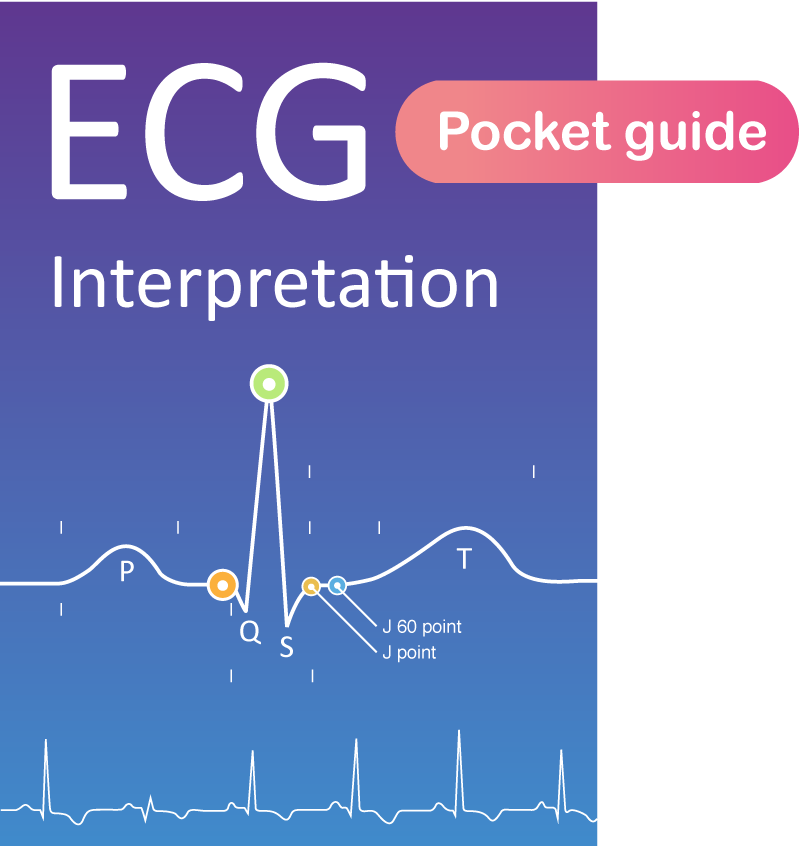Back to Book/course

## Clinical Echocardiography

0% Complete
0/0 Steps
Section 4, Chapter 5
In Progress

# Left ventricular mass and volume (size)

Chapter contents

Section Progress
0% Complete

## Size and mass of the left ventricle

Left ventricular mass is a flawed proxy for ventricular systolic function and load. The mass is, however, an important parameter in the assessment of ventricular hypertrophy and cardiomyopathy. Numerous formulas have been developed to approximate ventricular mass. Most formulas are simple mathematical equations, included in all ultrasound systems, and assume that the geometry of the left ventricle is normal.

massLV = 1.05 (masstotal – masscavity)
LV = left ventricle; 1.05 = mycoardial mass constant.

### Left ventricular hypertrophy (LVH)

A diagnosis of left ventricular hypertrophy is based on total left ventricular mass, which can be calculated by obtaining the measurements shown in Figure 1. The ultrasound system automatically calculates RWT (Relative Weight Thickness), provided that the patient’s weight, height and sex are entered. RWT is a measure of the type of hypertrophy.

Generally, hypertrophy is defined as wall thickness exceeding 12 mm. Thus, wall thickness >12 mm should raise suspicion of hypertrophy.

error: Contact us for permission to use contents. Permission will be granted for non-profit sites.